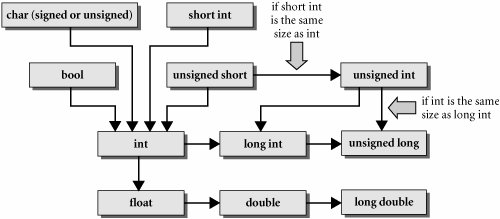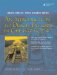# Standard Expression Conversions

Expression conversions, including implicit type conversions through promotion or demotion, and explicit casting through a variety of casting mechanisms are discussed in this section.

Suppose x and y are numeric variables. An expression of the form x op y has both a value and a type. When this expression is evaluated, temporary copies of x and y are used. If x and y have different types, the one with the shorter type may need to be converted (widened) before the operation can be performed. An implicit conversion of a number that preserves its value is called a promotion.

Automatic Expression Conversion Rules for x op y

1. Any bool, char, signed char, unsigned char, enum, short int, or unsigned short int is promoted to int. This is called an integral promotion.
2. If, after the first step, the expression is of mixed type, then the operand of smaller type is promoted to that of the larger type, and the value of the expression has that type.
3. The hierarchy of types is indicated by the arrows in Figure 19.1.

Figure 19.1. Hierarchy of basic typesWe note that the relationship between unsigned and long depends on the implementation. For example, on a system that implements int with the same number of bytes as long, it would not be possible to promote unsigned to long, so the promotion process would bypass long and promote unsigned to unsigned long. Now assume that we have the following declarations:

```double d;
int i;
```

In general, a promotion such as d = i; will be well behaved. An assignment that causes a demotion such as i = d; will result in a loss of information. Assuming the compiler permits the assignment, the fractional part of d would be discarded. Example 19.2 demonstrates some of the conversions we have discussed.

Example 19.2. src/mixed-types.cpp

 ```#include using namespace std; int main() { int i, j = 88; double d = 12314.8723497; cout << "initially d = " << d << " and j = " << j << endl; cout << "The sum is: " << d + i << endl; i = d; cout << "after demoting d, i = " << i << endl; d = j; cout << "after promoting j, d = " << d << endl; } ```

Here is the compile and run.

```src> g++ mixed-types.cpp
mixed-types.cpp: In function 'int main()':
mixed-types.cpp:10: warning: converting to 'int' from 'double'
src> ./a.out
initially d = 12314.9 and j = 88
The sum is: 1.34527e+08
after demoting d, i = 12314
after promoting j, d = 88
src>
```

Exercise: Standard Expression Conversions

 Assume that we have the following declarations: ```double d = 123.456; int i = 789, j = -1; uint k = 10; ```   What is the type and what is the value of d + i? What is the type and what is the value of j + k? What happens with a promotion such as d = i;? What happens with a demotion such as i = d;?An Introduction to Design Patterns in C++ with Qt 4
ISBN: 0131879057
EAN: 2147483647
Year: 2004
Pages: 268